Скачать презентацию Phonons electron-phonon coupling Claudia Ambrosch-Draxl Department für

b7359be6b8237f5740c5a51e47bc8262.ppt

• Количество слайдов: 33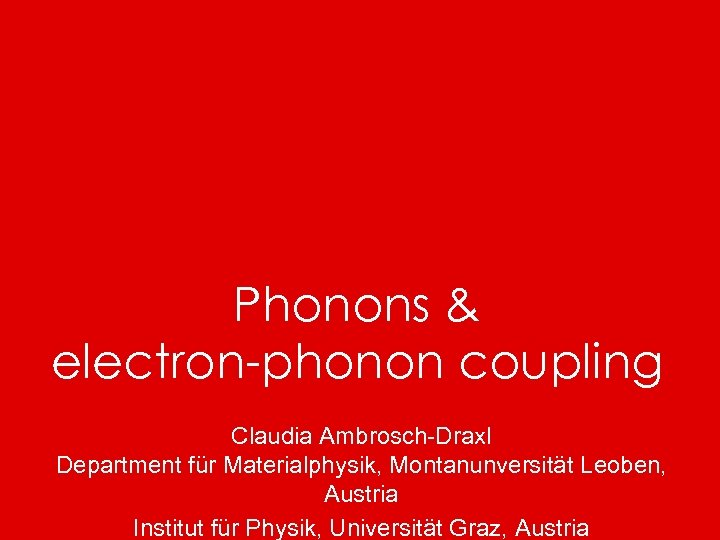Phonons & electron-phonon coupling Claudia Ambrosch-Draxl Department für Materialphysik, Montanunversität Leoben, Austria Institut für Physik, Universität Graz, Austria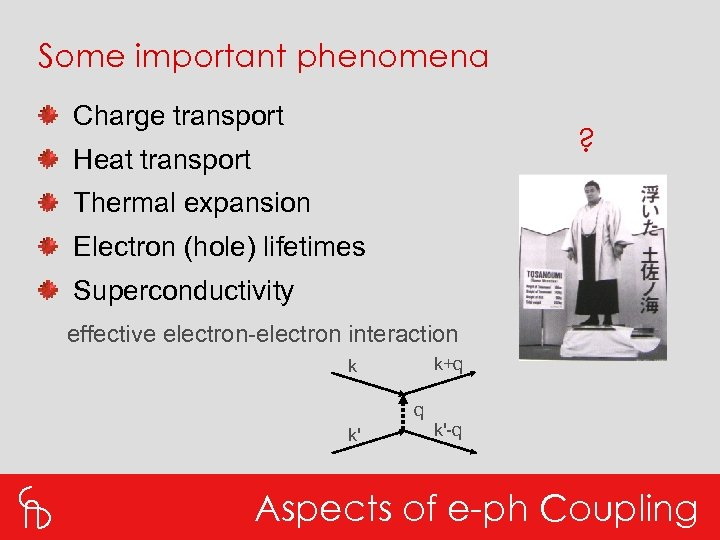Some important phenomena Charge transport ? Heat transport Thermal expansion Electron (hole) lifetimes Superconductivity effective electron-electron interaction k+q k q k' k'-q Aspects of e-ph Coupling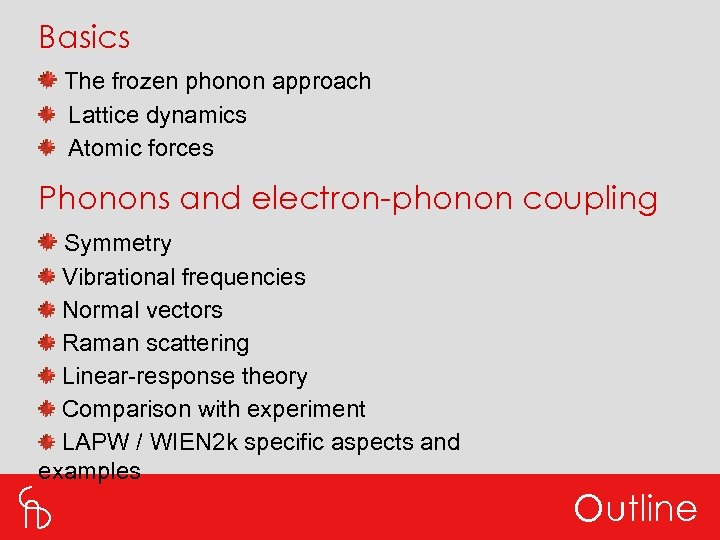Basics The frozen phonon approach Lattice dynamics Atomic forces Phonons and electron-phonon coupling Symmetry Vibrational frequencies Normal vectors Raman scattering Linear-response theory Comparison with experiment LAPW / WIEN 2 k specific aspects and examples Outline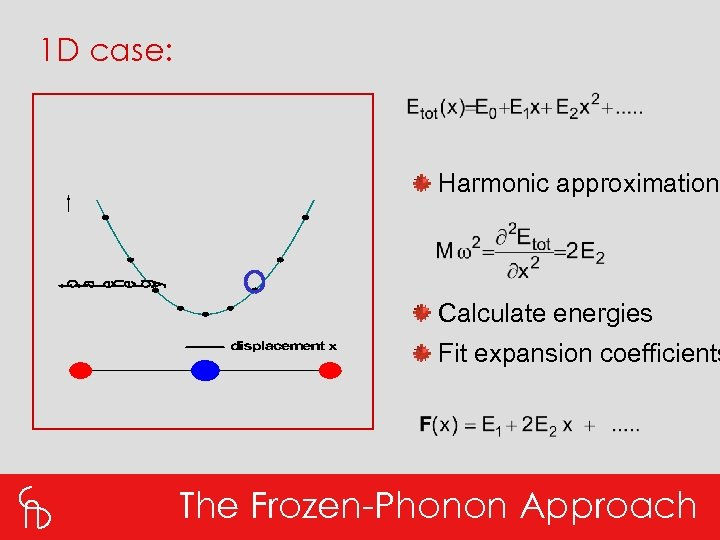1 D case: Harmonic approximation Calculate energies Fit expansion coefficients The Frozen-Phonon Approach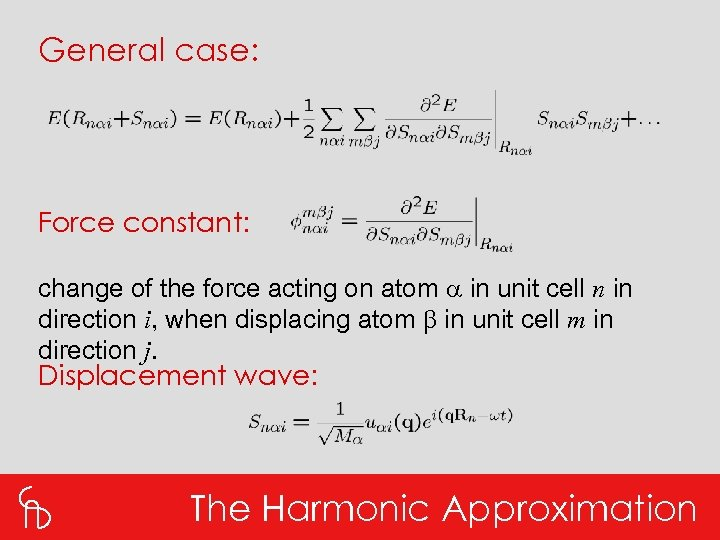General case: Force constant: change of the force acting on atom a in unit cell n in direction i, when displacing atom b in unit cell m in direction j. Displacement wave: The Harmonic Approximation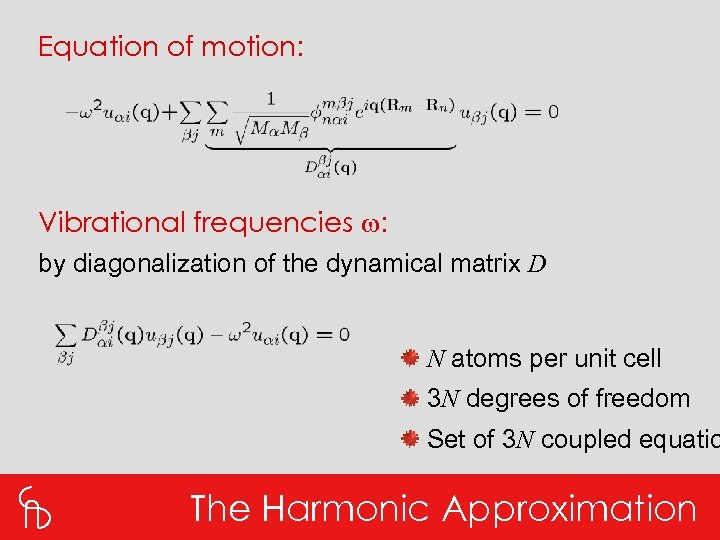Equation of motion: Vibrational frequencies w: by diagonalization of the dynamical matrix D N atoms per unit cell 3 N degrees of freedom Set of 3 N coupled equatio The Harmonic Approximation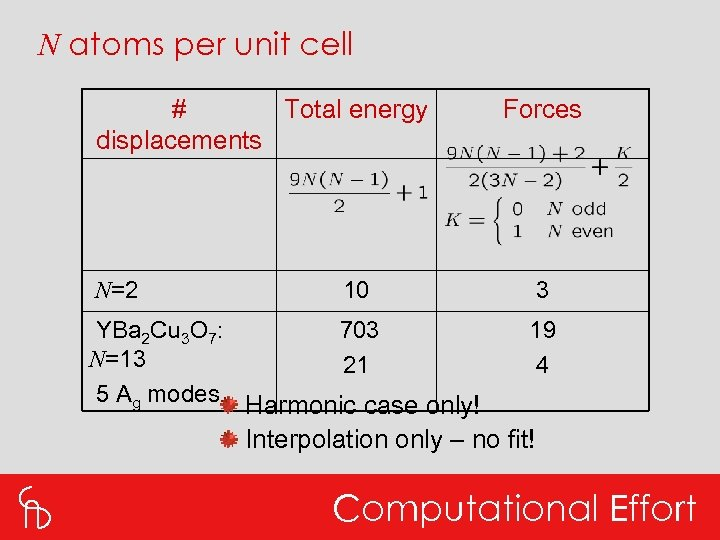N atoms per unit cell # Total energy displacements Forces N=2 10 3 YBa 2 Cu 3 O 7: N=13 5 Ag modes 703 21 19 4 Harmonic case only! Interpolation only – no fit! Computational Effort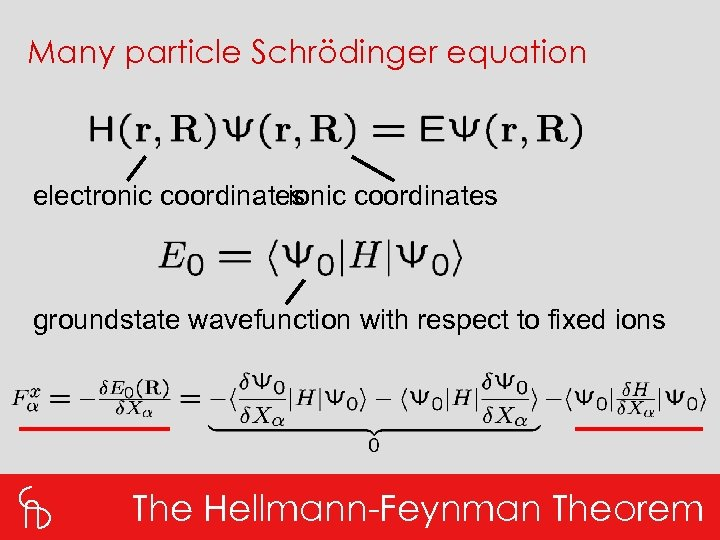Many particle Schrödinger equation electronic coordinates ionic coordinates groundstate wavefunction with respect to fixed ions The Hellmann-Feynman Theorem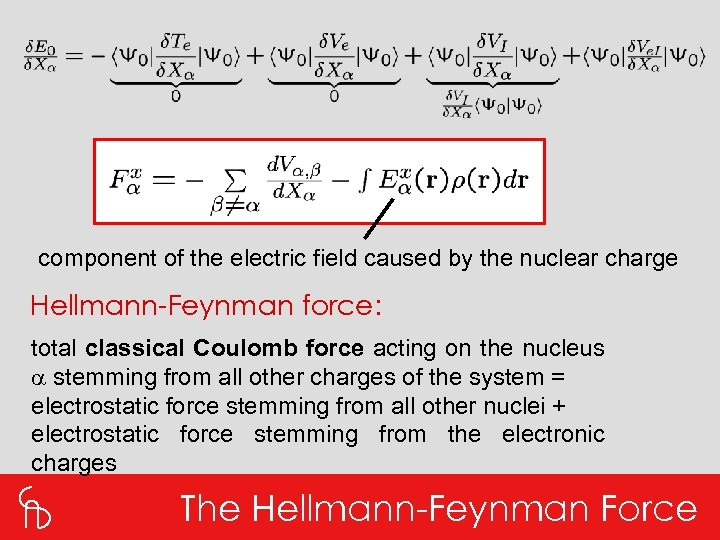component of the electric field caused by the nuclear charge Hellmann-Feynman force: total classical Coulomb force acting on the nucleus a stemming from all other charges of the system = electrostatic force stemming from all other nuclei + electrostatic force stemming from the electronic charges The Hellmann-Feynman Force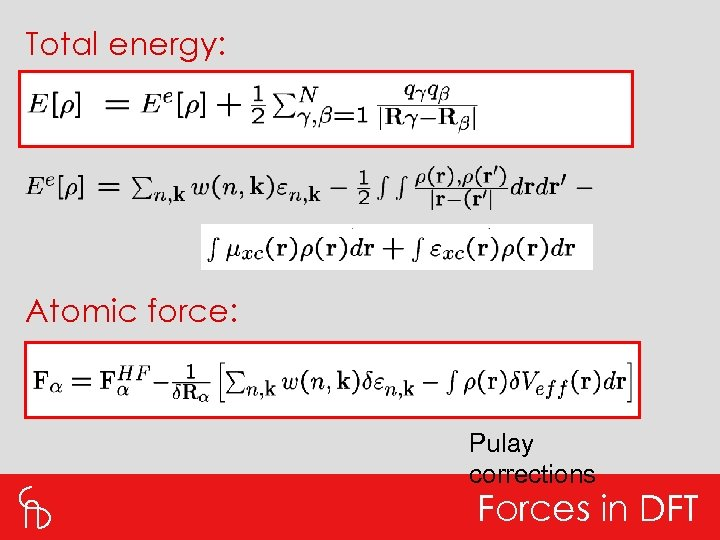Total energy: Atomic force: Pulay corrections Forces in DFT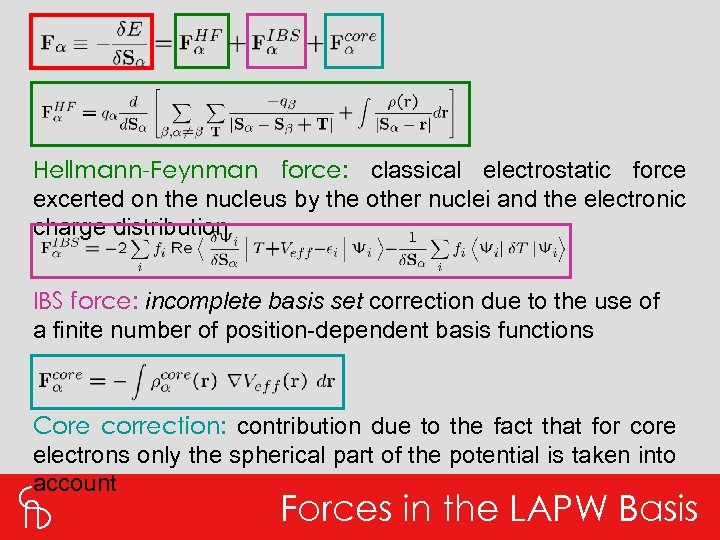Hellmann-Feynman force: classical electrostatic force excerted on the nucleus by the other nuclei and the electronic charge distribution IBS force: incomplete basis set correction due to the use of a finite number of position-dependent basis functions Core correction: contribution due to the fact that for core electrons only the spherical part of the potential is taken into account Forces in the LAPW Basis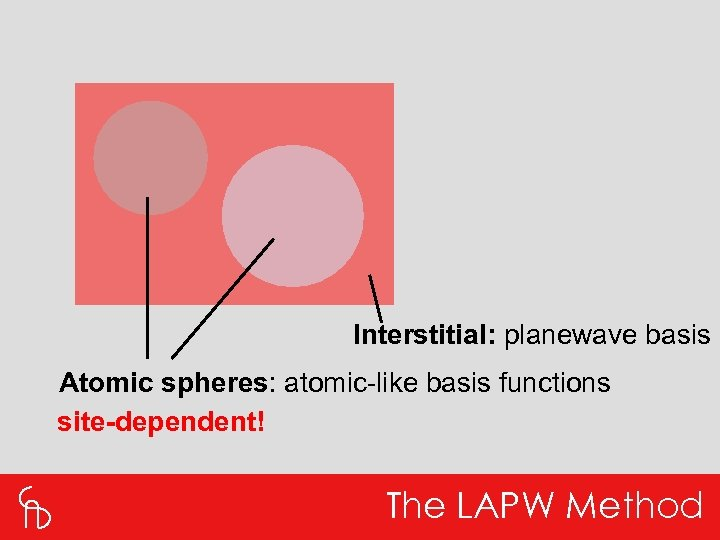Interstitial: planewave basis Atomic spheres: atomic-like basis functions site-dependent! The LAPW Method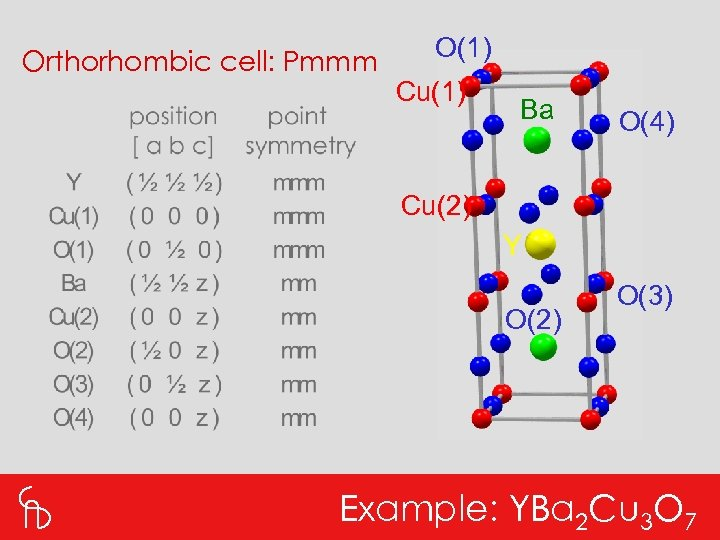Orthorhombic cell: Pmmm O(1) Cu(1) Ba O(4) Cu(2) Y O(2) O(3) Example: YBa 2 Cu 3 O 7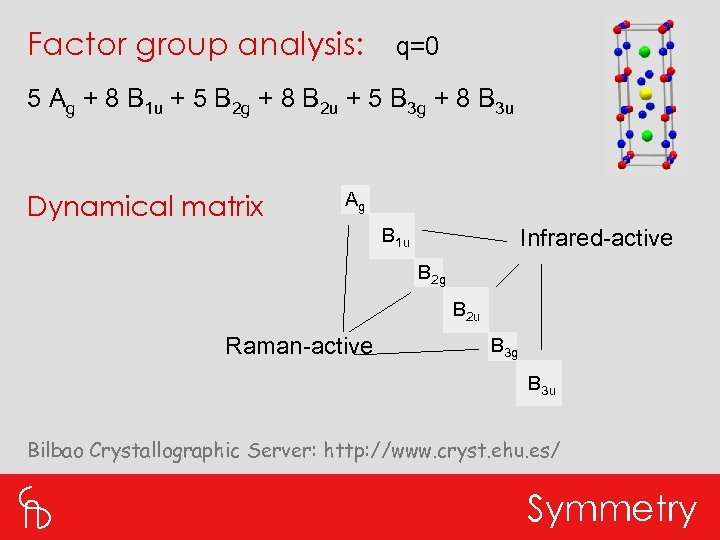Factor group analysis: q=0 5 Ag + 8 B 1 u + 5 B 2 g + 8 B 2 u + 5 B 3 g + 8 B 3 u Dynamical matrix Ag B 1 u Infrared-active B 2 g B 2 u Raman-active B 3 g B 3 u Bilbao Crystallographic Server: http: //www. cryst. ehu. es/ Symmetry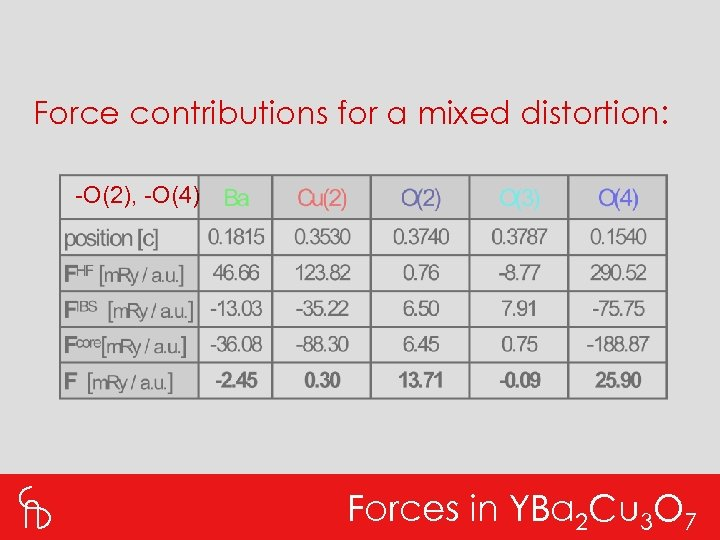Force contributions for a mixed distortion: -O(2), -O(4) Forces in YBa 2 Cu 3 O 7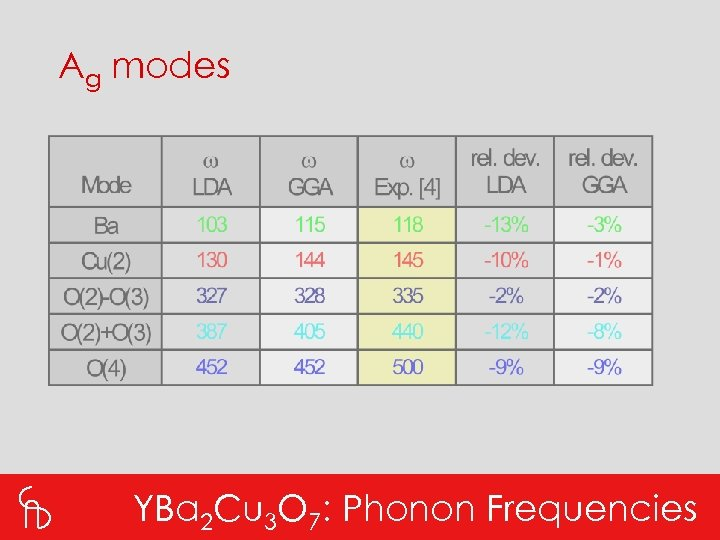Ag modes YBa 2 Cu 3 O 7: Phonon Frequencies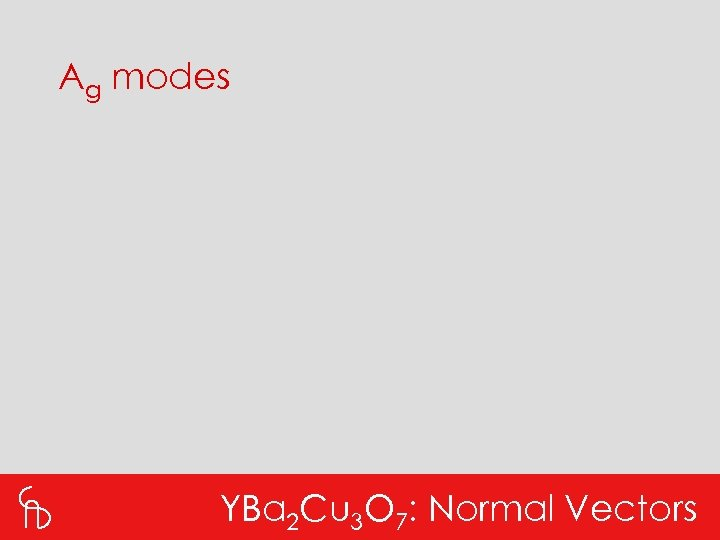Ag modes YBa 2 Cu 3 O 7: Normal Vectors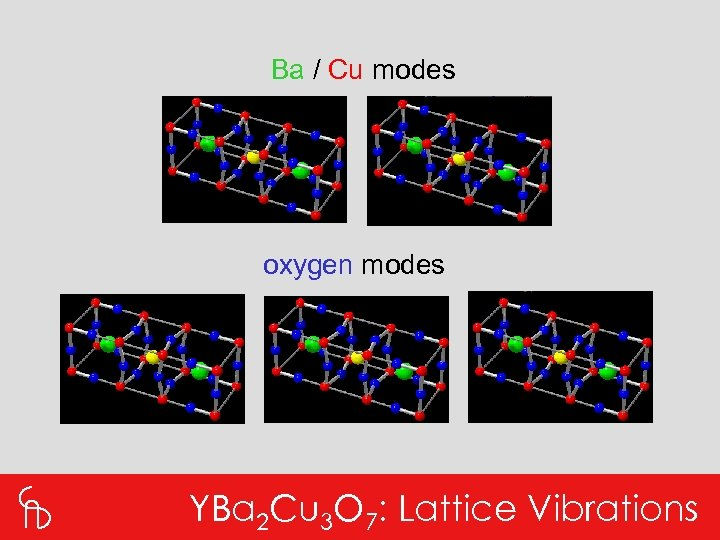Ba / Cu modes oxygen modes YBa 2 Cu 3 O 7: Lattice Vibrations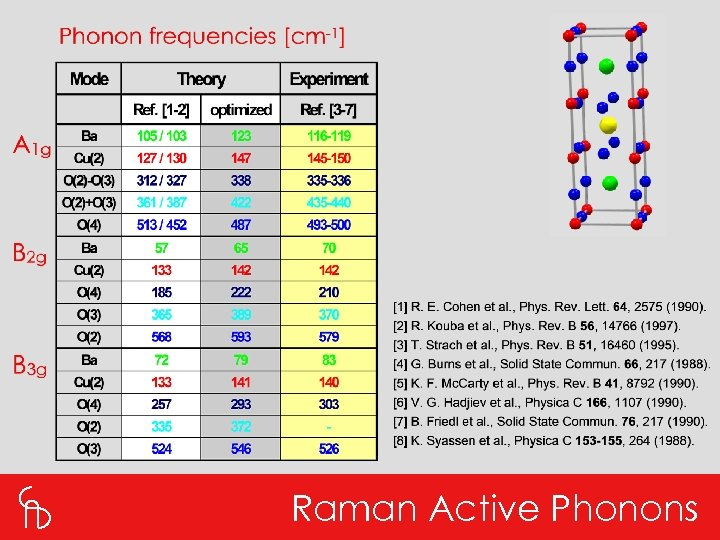Raman Active Phonons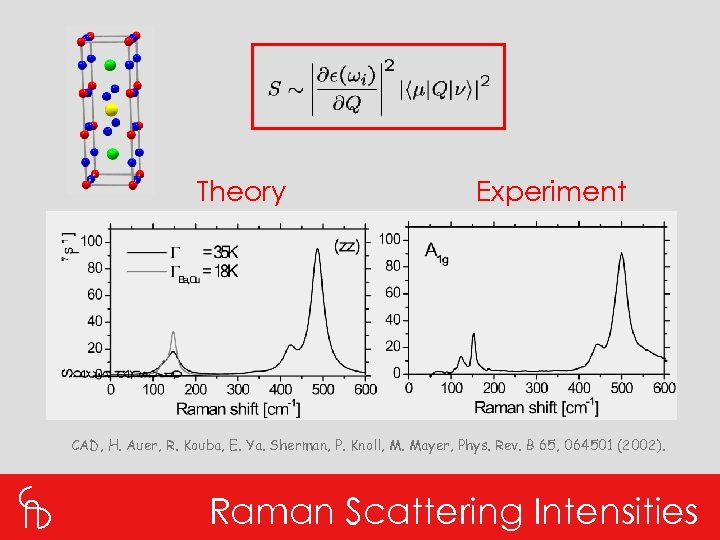Theory Experiment CAD, H. Auer, R. Kouba, E. Ya. Sherman, P. Knoll, M. Mayer, Phys. Rev. B 65, 064501 (2002). Raman Scattering IntensitiesO(4) mode Probing e-ph Coupling Strength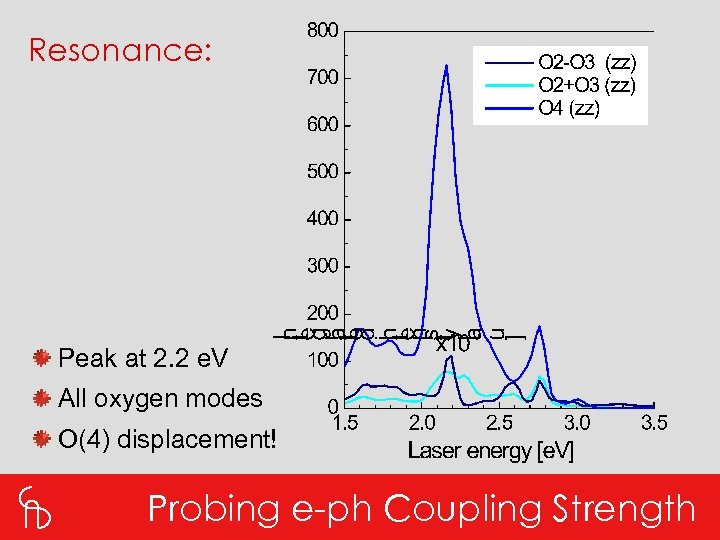Resonance: Peak at 2. 2 e. V All oxygen modes O(4) displacement! Probing e-ph Coupling Strength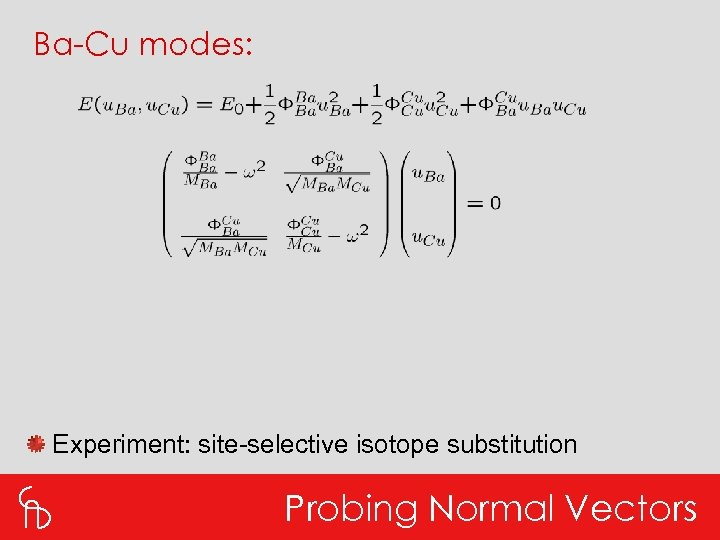Ba-Cu modes: Experiment: site-selective isotope substitution Probing Normal Vectors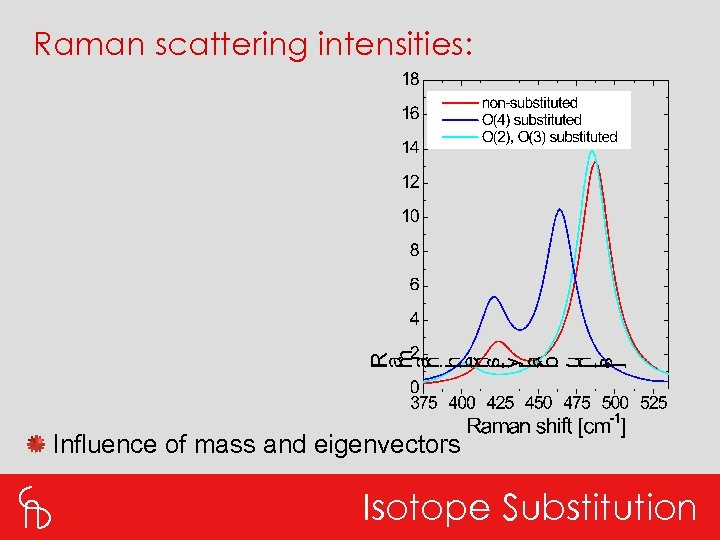Raman scattering intensities: Influence of mass and eigenvectors Isotope Substitution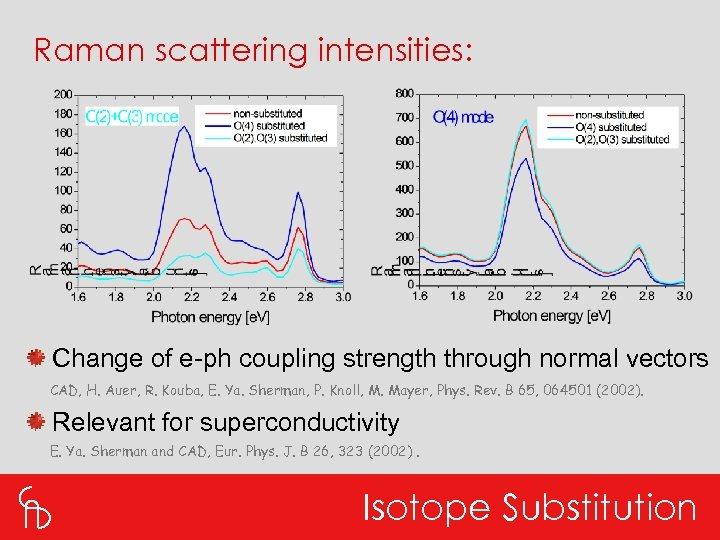Raman scattering intensities: Change of e-ph coupling strength through normal vectors CAD, H. Auer, R. Kouba, E. Ya. Sherman, P. Knoll, M. Mayer, Phys. Rev. B 65, 064501 (2002). Relevant for superconductivity E. Ya. Sherman and CAD, Eur. Phys. J. B 26, 323 (2002). Isotope Substitution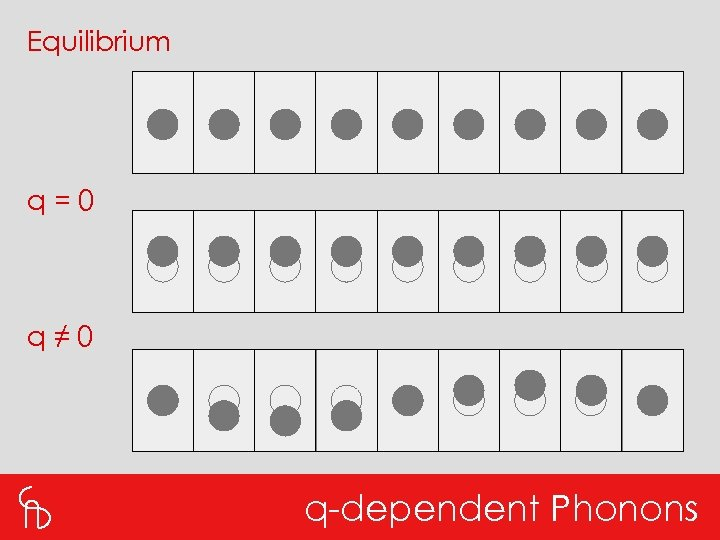Equilibrium q=0 q≠ 0 q-dependent Phonons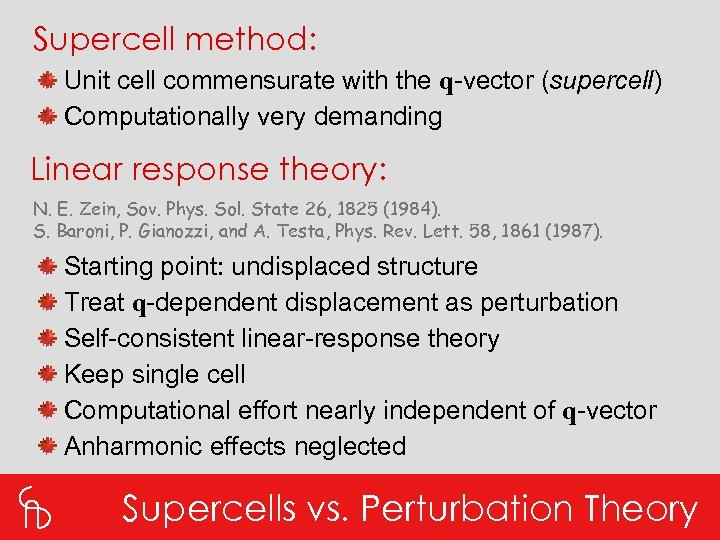Supercell method: Unit cell commensurate with the q-vector (supercell) Computationally very demanding Linear response theory: N. E. Zein, Sov. Phys. Sol. State 26, 1825 (1984). S. Baroni, P. Gianozzi, and A. Testa, Phys. Rev. Lett. 58, 1861 (1987). Starting point: undisplaced structure Treat q-dependent displacement as perturbation Self-consistent linear-response theory Keep single cell Computational effort nearly independent of q-vector Anharmonic effects neglected Supercells vs. Perturbation Theory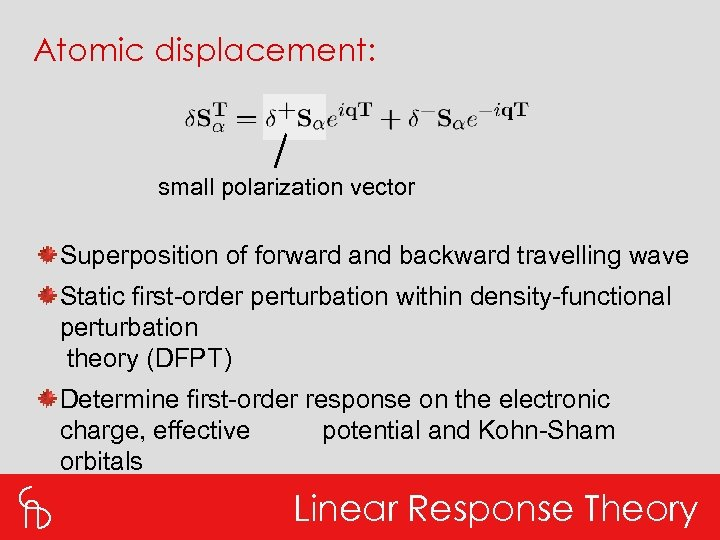Atomic displacement: small polarization vector Superposition of forward and backward travelling wave Static first-order perturbation within density-functional perturbation theory (DFPT) Determine first-order response on the electronic charge, effective potential and Kohn-Sham orbitals Linear Response Theory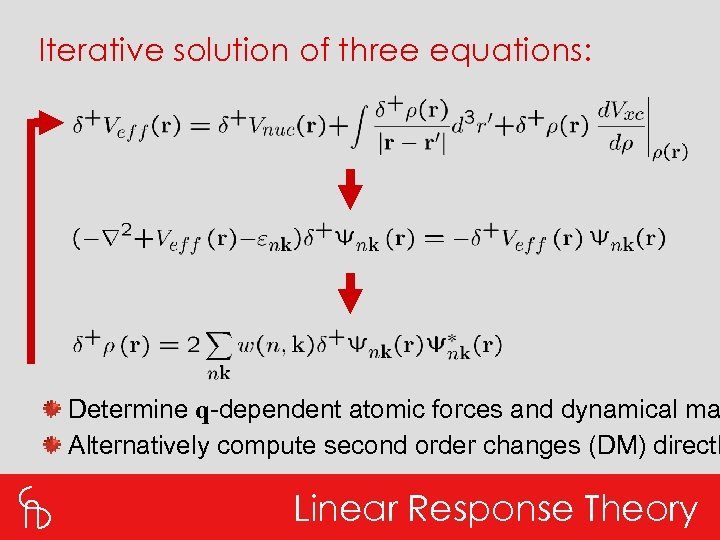Iterative solution of three equations: Determine q-dependent atomic forces and dynamical ma Alternatively compute second order changes (DM) directl Linear Response Theory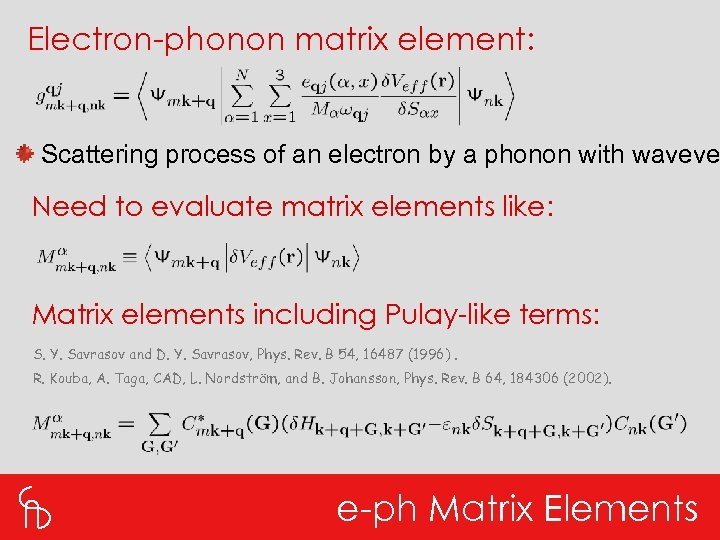Electron-phonon matrix element: Scattering process of an electron by a phonon with waveve Need to evaluate matrix elements like: Matrix elements including Pulay-like terms: S. Y. Savrasov and D. Y. Savrasov, Phys. Rev. B 54, 16487 (1996). R. Kouba, A. Taga, CAD, L. Nordström, and B. Johansson, Phys. Rev. B 64, 184306 (2002). e-ph Matrix Elements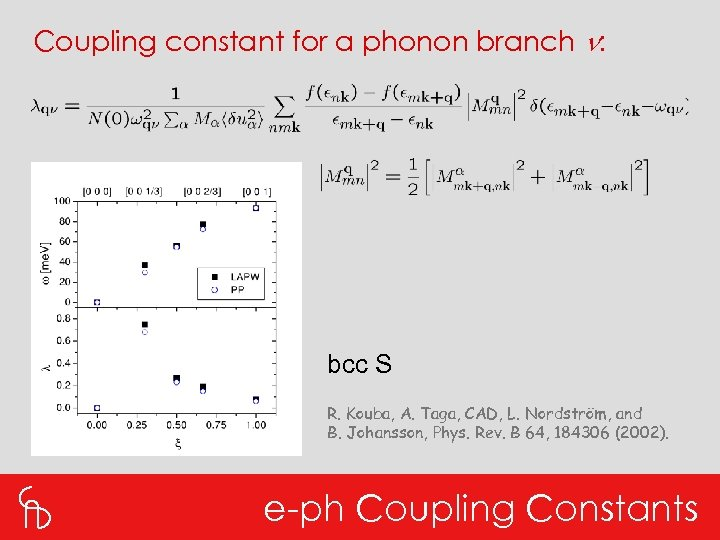Coupling constant for a phonon branch n: bcc S R. Kouba, A. Taga, CAD, L. Nordström, and B. Johansson, Phys. Rev. B 64, 184306 (2002). e-ph Coupling Constants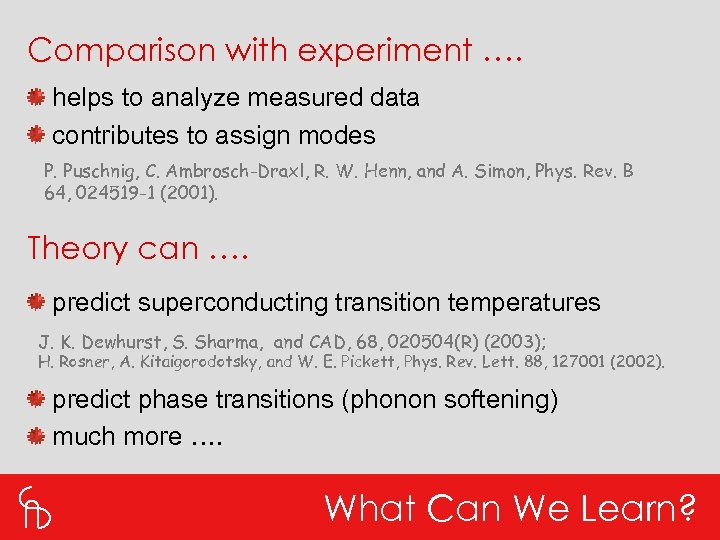Comparison with experiment …. helps to analyze measured data contributes to assign modes P. Puschnig, C. Ambrosch-Draxl, R. W. Henn, and A. Simon, Phys. Rev. B 64, 024519 -1 (2001). Theory can …. predict superconducting transition temperatures J. K. Dewhurst, S. Sharma, and CAD, 68, 020504(R) (2003); H. Rosner, A. Kitaigorodotsky, and W. E. Pickett, Phys. Rev. Lett. 88, 127001 (2002). predict phase transitions (phonon softening) much more …. What Can We Learn?Thank you for your attention!